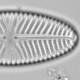## REDFIT & false alarms

REDFIT is a useful tool for palaeoecologists who like to test their data for periodicities as it uses the Lomb-Scargle Fourier transform which tolerates unequal time intervals and so avoids the problems inherent in interpolating data to equal intervals. Several of the papers reporting the imprint of solar-forcing on palaeoecological data used REDFIT, and I have been struck by how many periodicities they find to be statistically significant. Time to investigate what is going on.

REDFIT is a Fortran-90 program which can be run from the command line with a configuration file. It gets a little tedious formatting the data and writing the configuration file, so I wrote some R code to do this and then import and plot the output.

```redfit<-function(x, program="d:/moreprograms/redfit/bin/rf35wp", tempdir="d:/temp", nsim=1000, mctest=T, ofac=4, hifac=1, n50=3, rhopre=-99, iwin=2){
#check data
stopifnot(ncol(x)==2, all(diff(x[,1])>=0))

#write data to temporary file
data.file<-paste(tempdir,"rfdata.dat", sep="/")
write.table(x,file=data.file, row.names=FALSE, col.names=FALSE)

#write configuration file - see redfit usage file for options
out.file<-paste(tempdir,"rfout.out", sep="/")
cfg.string<-paste("&cfg \nfnin='",data.file,"', \nfnout='",out.file,"', \nnsim=",nsim,", \nmctest=",mctest,", \nofac=",ofac,", \nhifac=",hifac,", \nn50=",n50,", \nrhopre=",rhopre,", \niwin=",iwin," \n/", sep="")

cfg.file<-paste(tempdir,"rfcfg.cfg", sep="/")
write(cfg.string, file=cfg.file)

#run redfit
system(paste(program, cfg.file))

res<-list()
crit.fa<-res\$redtop
res\$crit.fa<-as.numeric(substr(crit.fa,49,100))
crit.fa.mc<-res\$redtop
res\$crit.fa.mc<-as.numeric(substr(crit.fa,56,100))

#return redfit object
class(res)<-"redfit"
res
}

plot.redfit<-function(x, legend=TRUE, ax.freq=FALSE, ax.at, th=TRUE, mc=FALSE, fal=1:4, ...){
use.col<-c(3)
if(th)use.col<-c(use.col,4)
if(mc)use.col<-c(use.col, 10+fal)
else use.col<-c(use.col, 6+fal)
matplot(x\$redfit\$Freq, x\$redfit[,use.col],type="l",xaxt="n",xlab="Period yrs",ylab="Spectral amplitude", ...)
if(ax.freq){
axis(side=1)
}else{
if(missing(ax.at))ax.at<-2^(1:floor(log(1/x\$redfit\$Freq,2)))
axis(side=1, at=1/ax.at, labels=ax.at)
}
if(legend) legend("topright", legend=colnames(x\$redfit)[use.col], lty=1:5, col=1:6)
}

identify.redfit<-function(x, ...){
identify(x\$redfit\$Freq, x\$redfit\$Gxx_corr, labels=round(1/x\$redfit\$Freq), ...)
}
```

Read the REDFIT usage guide to understand all the options for running the code.

I’m going to test this with some random data. REDFIT takes several seconds to run – the Monte Carlo procedure takes some time.

```x<-data.frame(1:100, as.vector(arima.sim(list(ar=.2), n=100)))
rdf<-redfit(x)
plot(rdf)
identify(rdf)
```

There is a statistically significant peak at about 2 yr (at p=0.95) and peaks at 3 and 6 yr that approach significance. But this is random data, there should not be any statistically significant peaks in the data, except by chance. Such peaks appear in many, perhaps most trials of random data. Panic. What have I not understood? Time to reread Schulz & Mudelsee (2002).

“We follow Thomson (1990) and select a false-alarm level of (1-1=/n)*100%, where n is the number of data points in each WOSA segment.”

Thomson (1990) makes it clear:

“It is important to remember that in typical time-series problems hundreds or thousands of uncorrelated estimates are being dealt with; consequently one will encounter numerous instances of the F-test giving what would normally be considered highly significant test values that, in actuality, will only be sampling fluctuations. A good rule-of-thumb is not to get excited by significance levels less than 1-1/N”

So it is the old familiar problem of multiple testing — when testing enough frequencies, some will appear statistically significant just by chance. With the random data used above, this rule-of-thumb sets the critical false alarm level to 98%, which is not exceeded. There is no evidence of significant periodicities in my random data. Relief.

None of the papers using REDFIT that I have looked at recently, including mine, give sufficient information to reconstruct what was done: the options used are not specified, and this critical false alarm is not discussed. I strongly suspect that most of the papers have not considered this critical false alarm, and so are interpreting periodicities before they have established that there are periodicities.Ecologist with interests in quantitative methods and palaeoenvironments
This entry was posted in EDA, Peer reviewed literature, R and tagged , . Bookmark the permalink.

### 3 Responses to REDFIT & false alarms

1.Kau Thirumalai says:

Excellent post – thank you! I had much difficulty trying to get REDFIT to work even with their MATLAB GUI…

•richard telford says:

I now use redfit from the R dplR package. It works very nicely.

I have some redfit related posts in the pipeline – stay tuned.